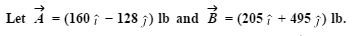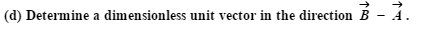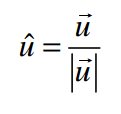# Statics: Dimensionless Unit Vector

• belvol16

## Homework Statement## Homework Equations## The Attempt at a Solution

So I began by subtracting.
(205-160)=55 i
(495+128)=623 j
Both of these vectors are in the positive direction. So if I divide the vector by its magnitude I should get an answer of 1 in the positive direction for both i and j.
The online book lists the answers as R hat = 0.0720 i + 0.997 j . I guess I'm not sure how the book got these answers. I thought unit vectors always had a magnitude of 1 and their purpose was to determine the direction of the vector.
Thank You!

So if I divide the vector by its magnitude I should get an answer of 1 in the positive direction for both i and j.
If each component had a magnitude of 1 then the vector would not be a unit vector!

The online book lists the answers as R hat = 0.0720 i + 0.997 j . I guess I'm not sure how the book got these answers. I thought unit vectors always had a magnitude of 1 and their purpose was to determine the direction of the vector.
The unit vector does have a magnitude of 1. Given those components, find the magnitude of the full vector. What do you get?

Minor point, but ##\vec{B} - \vec{A}## has units of lbs, and so would a unit vector in this direction. I'm puzzled by the instruction to "find a dimensionless unit vector" in this direction.

Minor point, but ##\vec{B} - \vec{A}## has units of lbs, and so would a unit vector in this direction. I'm puzzled by the instruction to "find a dimensionless unit vector" in this direction.
If you divide by the magnitude (also in lbs) you can get a dimensionless direction vector.

If you divide by the magnitude (also in lbs) you can get a dimensionless direction vector.
OK, that makes sense. Objection withdrawn...